Maths-
General
Easy

Question

# A and B can do a piece of work in 18 days; B and C in 24 days and A and C in 36 days. In what time can they do it, all working together?Hint:

## The correct answer is: 8 hours.

### Let’s say the total work is represented as W and let’s say the work done by A in 1 day is WA, work done by B in 1 day is WB andwork done by C in 1 day is WCStep 1 of 3:It is given that A and B can do a piece of work in 18 days. So we can writeWA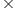18 + WB18 = WWA  + WB =     …….(1)It is given that B and C  can do a piece of work in 24 days. So we can writeWB24 + WC24 = WWB + WC =     …….(2)It is given that A and C  can do a piece of work in 36 days. So we can writeWA36 + WC36 = WWA + WC =      …….(3)Step 2 of 3:Now, adding equations (1), (2) and (3)2(WA  + WB + WC )=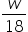+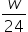+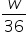WA  + WB + WC =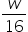……(4)Step 3 of 3:Let’s say the time taken by all of them to complete the work is t. So we can writeWAt + WBt + WCt = Wt(WA  + WB + WC) = WUsing equation (4)t= Wt = 16 daysFinal Answer: Hence, the time taken by all of them to complete the work is 8 hours.#### With Turito Foundation.#### Get an Expert Advice From Turito.